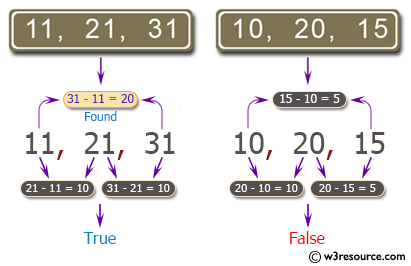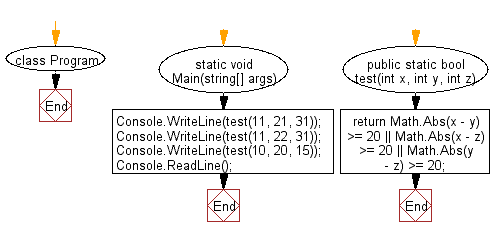﻿ C# - 3 integers, if one is 20 or more less than the others# C# Sharp Basic Algorithm Exercises: Check three given integers and return true if one of them is 20 or more less than one of the others

## C# Sharp Basic Algorithm: Exercise-51 with Solution

Write a C# Sharp program to check three given integers and return true if one of them is 20 or more less than one of the others.

Pictorial Presentation:Sample Solution:-

C# Sharp Code:

``````using System;
using System.Linq;
namespace exercises
{
class Program
{
static void Main(string[] args)
{
Console.WriteLine(test(11, 21, 31));
Console.WriteLine(test(11, 22, 31));
Console.WriteLine(test(10, 20, 15));
}
public static bool test(int x, int y, int z)
{
return Math.Abs(x - y) >= 20 || Math.Abs(x - z) >= 20 ||
Math.Abs(y - z) >= 20;
}
}
}
```
```

Sample Output:

```True
True
False```

Flowchart:C# Sharp Code Editor:

Improve this sample solution and post your code through Disqus

What is the difficulty level of this exercise?

Test your Programming skills with w3resource's quiz.

﻿